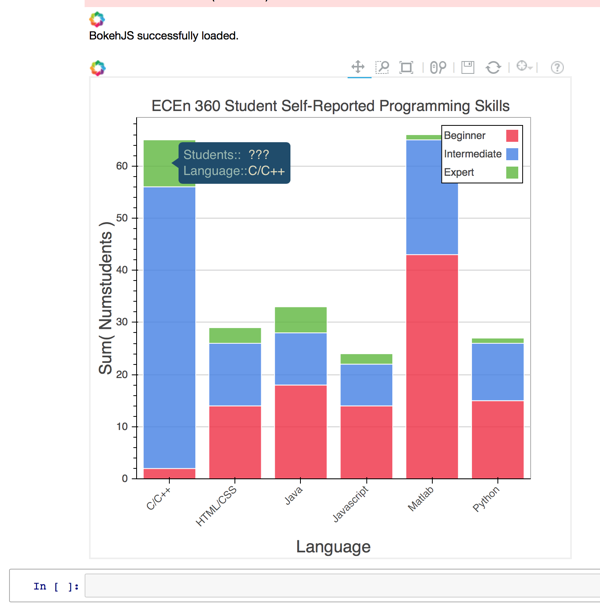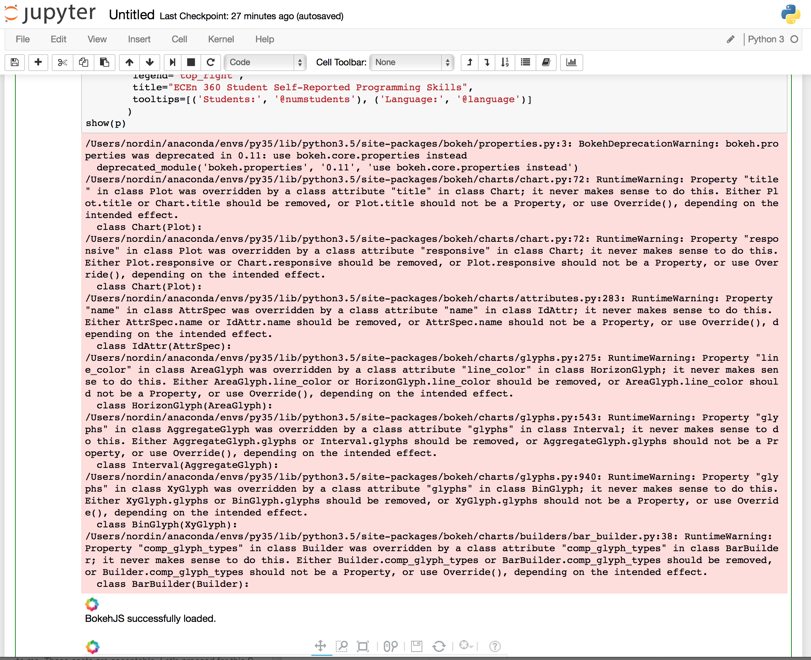# Tooltips problem with simple stacked bar chart

I’m running Bokeh 0.11.0 on Mac OS X 10.10.4 with Python 3.4. I have the following code in a Jupyter Notebook:

from bokeh.charts import Bar, show, output_notebook

import pandas as pd

output_notebook()

data_dict = { ‘numstudents’ : [43, 22, 1,

2, 54, 9,

18, 10, 5,

14, 12, 3,

15, 11, 1,

14, 8, 2],

‘language’ : [‘Matlab’,‘Matlab’,‘Matlab’,

‘C/C++’,‘C/C++’,‘C/C++’,

‘Java’,‘Java’,‘Java’,

‘HTML/CSS’,‘HTML/CSS’,‘HTML/CSS’,

‘Python’,‘Python’,‘Python’,

‘Javascript’,‘Javascript’,‘Javascript’],

‘skill_level’ : [‘Beginner’,‘Intermediate’,‘Expert’,

‘Beginner’,‘Intermediate’,‘Expert’,

‘Beginner’,‘Intermediate’,‘Expert’,

‘Beginner’,‘Intermediate’,‘Expert’,

‘Beginner’,‘Intermediate’,‘Expert’,

‘Beginner’,‘Intermediate’,‘Expert’]

}

data_df = pd.DataFrame(data_dict)

p = Bar(data_df,

values=‘numstudents’,

label=‘language’,

stack=‘skill_level’,

legend=‘top_right’,

title=“ECEn 360 Student Self-Reported Programming Skills”,

tooltips=[(‘Students:’, ‘@numstudents’), (‘Language:’, ‘@language’)]

)

show§

The plot builds and displays just fine. The problem I am having is with the tooltip information as illustrated in the upper left of the plot image below. Note that to the right of “Students:” is “???” rather than the number of students at that skill level for that language (in this case it should be the number 9). Why does this not work? My understanding from the documentation is that ‘@numstudents’ should refer to the column ‘numstudents’, but the tooltip doesn’t pick up the value from the column as it should, and as it correctly does in the case of the ‘language’ column.

Thanks,

GregBy the way, when executing the above code a massive set of warnings are emitted as shown below. I’ve gotten to where I just ignore them, but it would probably be better that they are addressed.I thought we'd rooted all those out. Can you make a GH issue with this screenshot?

Thanks,

Bryan

···

On Jan 9, 2016, at 12:30 AM, Greg Nordin <[email protected]> wrote:

By the way, when executing the above code a massive set of warnings are emitted as shown below. I've gotten to where I just ignore them, but it would probably be better that they are addressed.

--
You received this message because you are subscribed to the Google Groups "Bokeh Discussion - Public" group.
To unsubscribe from this group and stop receiving emails from it, send an email to [email protected].
To post to this group, send email to [email protected].
To view this discussion on the web visit https://groups.google.com/a/continuum.io/d/msgid/bokeh/66c11d41-7688-4ccd-a4b6-1a22633e5a1e%40continuum.io.

At a glance, that looks fine. I recall some case before where "???" was showing up, I will see if I can dig up the reference. But this might also be a hover + Bar bug or problem, in the mean time I'd suggest a GH issue, so some other people could look at it and track it more easily.

Thanks,

Bryan

···

On Jan 9, 2016, at 12:17 AM, Greg Nordin <[email protected]> wrote:

I'm running Bokeh 0.11.0 on Mac OS X 10.10.4 with Python 3.4. I have the following code in a Jupyter Notebook:

from bokeh.charts import Bar, show, output_notebook
import pandas as pd

output_notebook()

data_dict = { 'numstudents' : [43, 22, 1,
2, 54, 9,
18, 10, 5,
14, 12, 3,
15, 11, 1,
14, 8, 2],
'language' : ['Matlab','Matlab','Matlab',
'C/C++','C/C++','C/C++',
'Java','Java','Java',
'HTML/CSS','HTML/CSS','HTML/CSS',
'Python','Python','Python',
'Javascript','Javascript','Javascript'],
'skill_level' : ['Beginner','Intermediate','Expert',
'Beginner','Intermediate','Expert',
'Beginner','Intermediate','Expert',
'Beginner','Intermediate','Expert',
'Beginner','Intermediate','Expert',
'Beginner','Intermediate','Expert']
}

data_df = pd.DataFrame(data_dict)

p = Bar(data_df,
values='numstudents',
label='language',
stack='skill_level',
legend='top_right',
title="ECEn 360 Student Self-Reported Programming Skills",
tooltips=[('Students:', '@numstudents'), ('Language:', '@language')]
)
show(p)

The plot builds and displays just fine. The problem I am having is with the tooltip information as illustrated in the upper left of the plot image below. Note that to the right of "Students:" is "???" rather than the number of students at that skill level for that language (in this case it should be the number 9). Why does this not work? My understanding from the documentation is that '@numstudents' should refer to the column 'numstudents', but the tooltip doesn't pick up the value from the column as it should, and as it correctly does in the case of the 'language' column.

Thanks,
Greg

--
You received this message because you are subscribed to the Google Groups "Bokeh Discussion - Public" group.
To unsubscribe from this group and stop receiving emails from it, send an email to [email protected].
To post to this group, send email to [email protected].

···

On Sunday, January 10, 2016 at 10:03:09 AM UTC-7, Bryan Van de ven wrote:

I thought we’d rooted all those out. Can you make a GH issue with this screenshot?

Thanks,

Bryan

On Jan 9, 2016, at 12:30 AM, Greg Nordin [email protected] wrote:

By the way, when executing the above code a massive set of warnings are emitted as shown below. I’ve gotten to where I just ignore them, but it would probably be better that they are addressed.

You received this message because you are subscribed to the Google Groups “Bokeh Discussion - Public” group.

To unsubscribe from this group and stop receiving emails from it, send an email to [email protected].

To post to this group, send email to [email protected].

To view this discussion on the web visit https://groups.google.com/a/continuum.io/d/msgid/bokeh/66c11d41-7688-4ccd-a4b6-1a22633e5a1e%40continuum.io.

···

On Sunday, January 10, 2016 at 10:07:22 AM UTC-7, Bryan Van de ven wrote:

At a glance, that looks fine. I recall some case before where “???” was showing up, I will see if I can dig up the reference. But this might also be a hover + Bar bug or problem, in the mean time I’d suggest a GH issue, so some other people could look at it and track it more easily.

Thanks,

Bryan

On Jan 9, 2016, at 12:17 AM, Greg Nordin [email protected] wrote:

I’m running Bokeh 0.11.0 on Mac OS X 10.10.4 with Python 3.4. I have the following code in a Jupyter Notebook:

from bokeh.charts import Bar, show, output_notebook

import pandas as pd

output_notebook()

data_dict = { ‘numstudents’ : [43, 22, 1,
2, 54, 9,
18, 10, 5,

``````                           14, 12, 3,
``````
``````                           15, 11, 1,
``````
``````                           14, 8, 2],
``````
``````          'language' : ['Matlab','Matlab','Matlab',
'C/C++','C/C++','C/C++',
'Java','Java','Java',
``````
``````                        'HTML/CSS','HTML/CSS','HTML/CSS',
``````
``````                        'Python','Python','Python',
``````
``````                        'Javascript','Javascript','Javascript'],
``````
``````          'skill_level' : ['Beginner','Intermediate','Expert',
'Beginner','Intermediate','Expert',
'Beginner','Intermediate','Expert',
``````
``````                           'Beginner','Intermediate','Expert',
``````
``````                           'Beginner','Intermediate','Expert',
``````
``````                           'Beginner','Intermediate','Expert']
``````
``````        }
``````

data_df = pd.DataFrame(data_dict)

p = Bar(data_df,
values=‘numstudents’,
label=‘language’,
stack=‘skill_level’,

``````    legend='top_right',
``````
``````    title="ECEn 360 Student Self-Reported Programming Skills",
``````
``````    tooltips=[('Students:', '@numstudents'), ('Language:', '@language')]
``````
``````   )
``````

show§

The plot builds and displays just fine. The problem I am having is with the tooltip information as illustrated in the upper left of the plot image below. Note that to the right of “Students:” is “???” rather than the number of students at that skill level for that language (in this case it should be the number 9). Why does this not work? My understanding from the documentation is that ‘@numstudents’ should refer to the column ‘numstudents’, but the tooltip doesn’t pick up the value from the column as it should, and as it correctly does in the case of the ‘language’ column.

Thanks,

Greg

You received this message because you are subscribed to the Google Groups “Bokeh Discussion - Public” group.

To unsubscribe from this group and stop receiving emails from it, send an email to [email protected].

To post to this group, send email to [email protected].